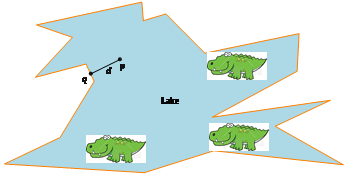# Answered Essay: Your friend has dropped you somewhere at a point p on Speedway Blvd. You need to walk to the nearest bus statio

Your friend has dropped you somewhere at a point p on Speedway Blvd. You need to walk to the nearest bus station, but you are not sure if the nearest station is east or west of your location, so you are not sure which direction to go.

Let d be the distance to the nearest bus station. Of course, d is not known to you in advance. Let w be the distance you walked (in the worst case) until reaching the station. The following algorithm has proposed.Figure 3: The lake. You have landed at p, and wish to swim to the shore. The nearest point of the shore is q. (credit: ClipPanda.com)

set s = 1 // 1 foot

while( not reached the bus station ) {

walk a distance of s East

walk a distance of s West

s = 3s

}

Assume d is much larger than 1 foot. Express w (as a function of d). Is it true that w = Ω(d) ? Is it true that w = O(d) ? What is the ratio w/d in the best case ? What is the ratio w/d in the worst case ? Prove your answers. Clarification The best case occurs when the bus station is positioned such that the ratio w/d is the smallest possible value. The worst case occurs when the bus station is positioned such that the ratio w/d is as large as possible.

You can put s = 1 if you want but I ve kept it as s

W = 2*(s + 3s + 3*3*S + 3*3*3*s + …. (3^k) * s )

where (3^k) * s >= d i.e the last step should be d or greater

3^k >= d/s

we can say

k = ceil (log3 (d/s) )

In assymptotic notation

k = log3 (d/s)

W is a geometric series with f(n) = 3*f(n-1)

Using gp sum, we get

W = 2 * s(3^(k+1) – 1) / (2)

= (3^(k+1) – 1) * s

= ~3^k * s …. for assymptotic notation

Plug the value of k, we have

W = 3 ^ log3 (d/s) * s

= d/s * s

= d

So, W = Θ(d) = O(d) = Ω(d)

W = (3^(k+1) – 1) * s

3^(k-1)*s > d >= 3^k * s

dmin = 3^(k-1) * s

dmax = 3^k * s

w/dmin = (3^(k+1) – 1) / 3^(k-1))

To maximize this we have to consider large values of k so we can ignore the -1 in numerator and we have

w/dmin = 3^(k+1) / 3^(k-1) = 9 … this is max ratio

w/dmax = (3^(k+1) – 1) / 3^(k)) …

To minimize this, we need to consider least values of k, so put k=0

w/dmax = 2 …. this is min ratioPages (550 words)
Approximate price: -

Help Me Write My Essay - Reasons:Best Online Essay Writing Service

We strive to give our customers the best online essay writing experience. We Make sure essays are submitted on time and all the instructions are followed.Our Writers are Experienced and Professional

Our essay writing service is founded on professional writers who are on stand by to help you any time.Free Revision Fo all Essays

Sometimes you may require our writers to add on a point to make your essay as customised as possible, we will give you unlimited times to do this. And we will do it for free.Timely Essay(s)

We understand the frustrations that comes with late essays and our writers are extra careful to not violate this term. Our support team is always engauging our writers to help you have your essay ahead of time.Customised Essays &100% Confidential

Our Online writing Service has zero torelance for plagiarised papers. We have plagiarism checking tool that generate plagiarism reports just to make sure you are satisfied.Try it now!

## Calculate the price of your order

Total price:
\$0.00

How it works?Fill in the order form and provide all details of your assignment.Proceed with the payment

Choose the payment system that suits you most.HOW OUR ONLINE ESSAY WRITING SERVICE WORKS

Let us write that nagging essay.By clicking on the "PLACE ORDER" button, tell us your requires. Be precise for an accurate customised essay. You may also upload any reading materials where applicable.Pick A & Writer

Our ordering form will provide you with a list of writers and their feedbacks. At step 2, its time select a writer. Our online agents are on stand by to help you just in case.Editing (OUR PART)

At this stage, our editor will go through your essay and make sure your writer did meet all the instructions.